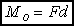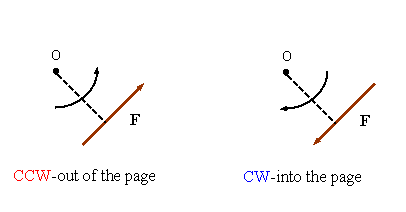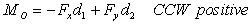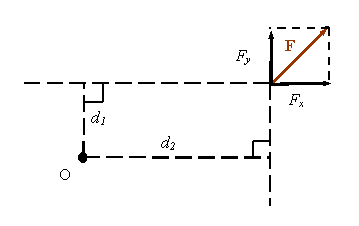Moment of a force: Part 1

The magnitude of the moment of a force:

MO: Magnitude of the moment of F around point O

d: Perpendicular distance from O to the line of action of FNote: moving a force along its line of action does not change its moment

Note:Direction of the moment in 2-D: The direction of the moment is given by the right hand rule: Counter Clockwise (CCW) is out of the page, Clockwise (CW) is into the page.Calculating the moment in 2-D using components: Moments add together as vectors. Select a positive direction (CCW or CW), then calculate each moment and add them using the proper sign for each term. For example: